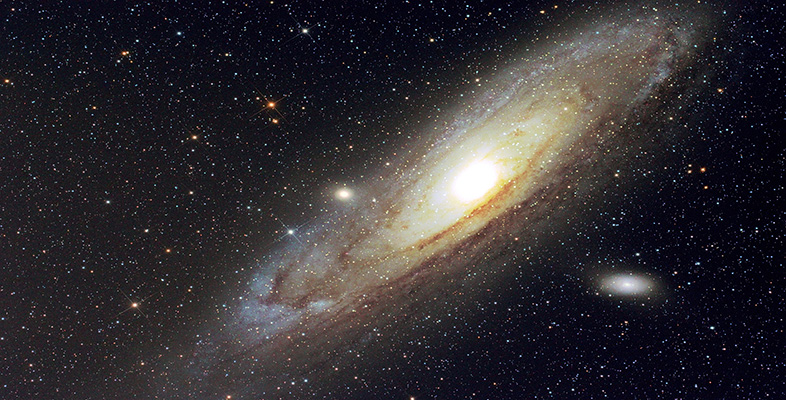Science, Maths & Technology

### Become an OU studentGalaxies, stars and planets

Start this free course now. Just create an account and sign in. Enrol and complete the course for a free statement of participation or digital badge if available.

# Answers to questions

Question 1 The Sun has a diameter of 1.4 million km which is 1400 000 km. The scale of the model is 1 cm to 5000 km. The diameter of the Sun in the model will therefore be 1400 000/5000 = 280 cm = 2.8 m. There is no fruit large enough to represent it in the model!

Question 2 The distance from the Sun to the Earth is 150 million km = 150 000 000 km = 150 000 000 000 m. Light travels at 300 million metres per second so it will take 150 000 000 000/300 000 000 = 500 seconds to travel from the Sun to the Earth. This is a tiny fraction of a year (which is over 30 million seconds) so a light-year is not an appropriate unit to measure distances within the Solar System.

Question 3 Star X is four times further away than star Y, so it will appear to be 42 = 4 × 4 = 16 times fainter than star Y.

Question 4 The composition of the crust is dominated by silicon and oxygen whereas the whole Earth has a smaller proportion of these elements indicating that the proportion of rocky material is lower overall. The proportion of iron is much higher in the whole Earth than in the crust, indicating that the iron is concentrated towards the centre.